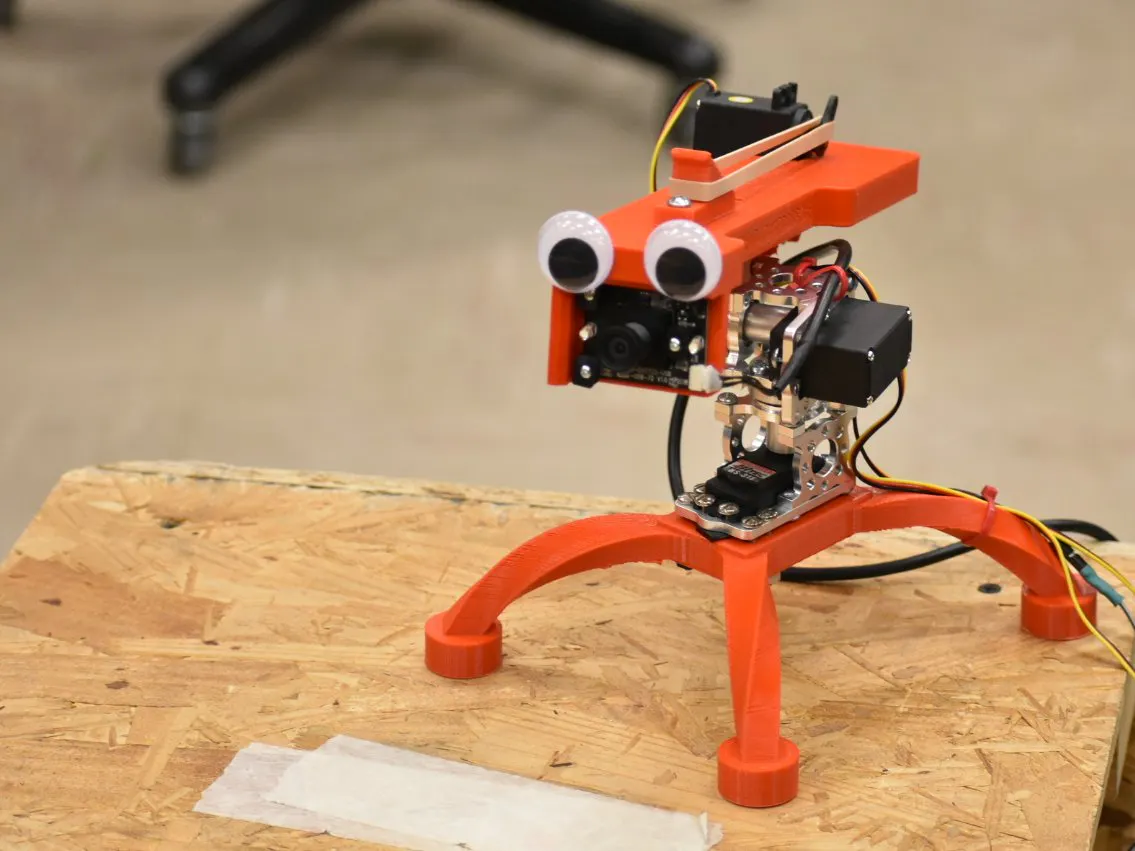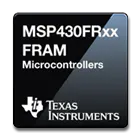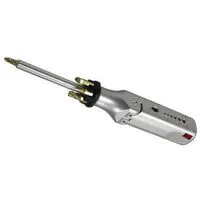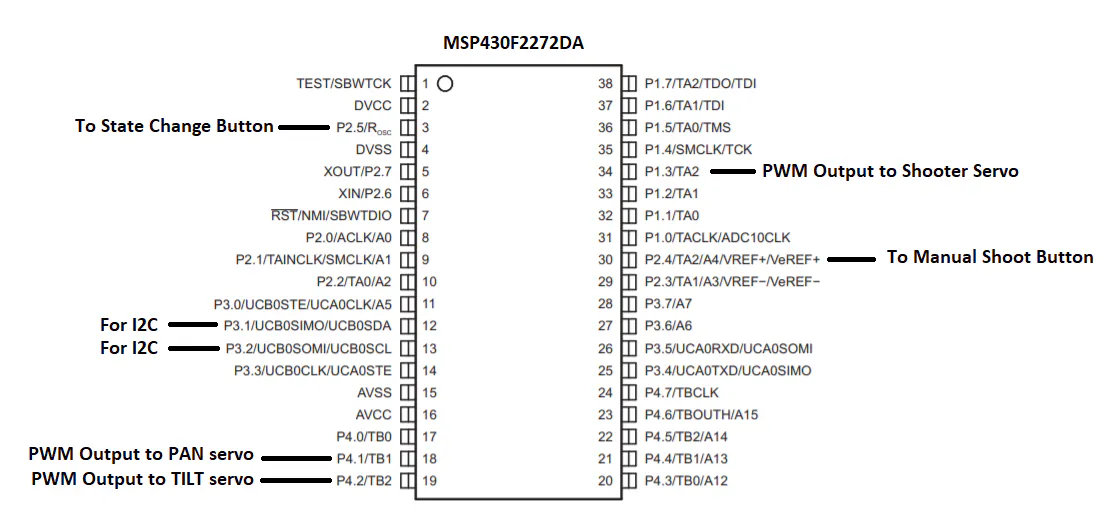# The Noot Shoot

A rubber band shooter that sits on top of a pan-tilt stage with computer vision abilities.

IntermediateShowcase (no instructions)20 hours509## Things used in this project

### Hardware componentsTexas Instruments MSP430 Microcontroller MSP430F2272 variant
×1
 Hitec HS-311
×3
 Orange Pi Lite
×1SparkFun Pushbutton switch 12mm
×2Machine Screw, M4
×10
 Rubber Bands A lot
×10

### Software apps and online servicesOpenCVTexas Instruments Code Composer Studio

### Hand tools and fabrication machines3D Printer (generic)Multitool, Screwdriver

## Schematics

### msp430_KRCJgnLg5F.PNG## Code

### shooter.c

C/C++
```/******************************************************************************
MSP430F2272 Project Creator 4.0

ME 461 - S. R. Platt
Fall 2010

Updated for CCSv4.2 Rick Rekoske 8/10/2011

Written by: Steve Keres
College of Engineering Control Systems Lab
University of Illinois at Urbana-Champaign
*******************************************************************************/

#include "msp430x22x2.h"
#include "UART.h"
#include "math.h"

char newprint = 0;
unsigned long timecnt = 0;

// i2c variables
unsigned char RXData  = {0, 0, 0, 0, 0, 0, 0, 0};
unsigned char TXData  = {0, 0, 0, 0, 0, 0, 0, 0};
int data_idx = 0;
long rxlong1 = 1000;
long rxlong2 = 1000;

// Controller Variables
int errorX = 0;
int errorY = 0;
int kpx = 3;
int kpy = 3;
long prevX = 3200;
long prevY = 3200;
long currX = 3200;
long currY = 3200;
long turnrateX = 1;
long turnrateY = 1;

// state machine variables
long btnPrsCnt = 0;
int btnPrs = 0;
long errorCnt = 0;

void main(void) {

WDTCTL = WDTPW + WDTHOLD;                 // Stop WDT

if (CALBC1_16MHZ ==0xFF || CALDCO_16MHZ == 0xFF) while(1);

DCOCTL = CALDCO_16MHZ;                      // Set uC to run at approximately 16 Mhz
BCSCTL1 = CALBC1_16MHZ;

//P1IN              Port 1 register used to read Port 1 pins setup as Inputs
P1SEL &= ~0xFF; // Set all Port 1 pins to Port I/O function
P1REN &= ~0xFF; // Disable internal resistor for all Port 1 pins
P1OUT &= ~0xFF;  // Initially set all Port 1 pins set as Outputs to zero
P1IFG &= ~0xFF;  // Clear Port 1 interrupt flags
P1IES &= ~0xFF; // If port interrupts are enabled a High to Low transition on a Port pin will cause an interrupt
P1IE &= ~0xFF; // Disable all port interrupts

P1DIR |= 0x8; // Set timer TA2
P1SEL |= 0x8; // Set timer TA2

//P2 IN
P2SEL &= 0x30; // Only 2.4 and 2.5
P2DIR &= 0x30; // P2.4 and P2.5 pin to input dir
P2REN = 0x30;  // P2.4 and 2.5 resistor enable
P2OUT = 0xC0;  // P2.4 and P2.5 pulled down
P2IES = 0xC0;
P2IFG = 0x00;  // clear flags
P2IE &= ~0xFF; // Disable all port interrupts

//P4IN Setting TB1 and TB2
P4DIR |= 0x6;
P4SEL |= 0x6;

//P3
P3SEL |= 0x06; // For i2c slave definition

UCB0CTL1 = UCSWRST +  UCSSEL_2;
UCB0CTL0 = UCSYNC + UCMODE_3;
UCB0I2COA = 0x25;
UCB0CTL1 &= ~UCSWRST;
IE2 |= UCB0RXIE;
IFG2 &= ~UCB0RXIFG; // This fixed the thing

// Timer A Config
TACCTL0 = 0; // Interrupt Disabled
TACCR0 = 40000;
TACTL = TASSEL_2 + MC_1 + ID_3;

// Timer B Config
TBCCTL0 = 0;
TBCCR0 = 40000; //20ms 16000*20=320000, 320000/8=40 000
TBCTL = TBSSEL_2 + MC_1 + ID_3;

// Servo Pan
TBCCTL1 = OUTMOD_7 + CLLD_1;
TBCCR1 = 3500;
// Servo Tilt
TBCCTL2 = OUTMOD_7 + CLLD_1;
TBCCR2 = 3750;
// Servo trigger
TACCTL2 = OUTMOD_7; //+ CLLD_1;
TACCR2 = 3200;

Init_UART(115200,1);  // Initialize UART for 115200 baud serial communication

_BIS_SR(GIE);   // Enable global interrupt

while(1) {

if(newmsg) {
//my_scanf(rxbuff,&var1,&var2,&var3,&var4);
newmsg = 0;
}

if (newprint)  {
//P1OUT ^= 0x1;     // Blink LED
//UART_printf("%d %d %d %d\n\r", (int)RXData, (int)RXData, (int)RXData, (int)RXData);
UART_printf("%ld %ld %d %d\n\r", rxlong1, rxlong2, (int)currX, (int)currY);
// UART_send(1,(float)timecnt/500);
newprint = 0;
}

}
}

// Timer A0 interrupt service routine
#pragma vector=TIMERA0_VECTOR
__interrupt void Timer_A (void)
{
timecnt++; // Keep track of time for main while loop.

if ((timecnt%500) == 0) {
//        newprint = 1;  // flag main while loop that .5 seconds have gone by.
}

}

// USCI Transmit ISR - Called when TXBUF is empty (ready to accept another character)
#pragma vector=USCIAB0TX_VECTOR
__interrupt void USCI0TX_ISR(void) {

if((IFG2&UCA0TXIFG) && (IE2&UCA0TXIE)) {        // USCI_A0 requested TX interrupt
if(printf_flag) {
if (currentindex == txcount) {
senddone = 1;
printf_flag = 0;
IFG2 &= ~UCA0TXIFG;
} else {
UCA0TXBUF = printbuff[currentindex];
currentindex++;
}
} else if(UART_flag) {
if(!donesending) {
UCA0TXBUF = txbuff[txindex];
if(txbuff[txindex] == 255) {
donesending = 1;
txindex = 0;
} else {
txindex++;
}
}
}

IFG2 &= ~UCA0TXIFG;
}

if((IFG2&UCB0RXIFG) && (IE2&UCB0RXIE)) {    // USCI_B0 RX interrupt occurs here for I2C
RXData[data_idx] = UCB0RXBUF;
TXData[data_idx] = RXData[data_idx];
data_idx++;
if (data_idx > 7) {
data_idx=0;
P1OUT ^= 0x04;
newprint = 1;
IE2 &= ~UCB0RXIE;
IE2 |= UCB0TXIE;
}

} else if ((IFG2&UCB0TXIFG) && (IE2&UCB0TXIE)) { // USCI_B0 TX interrupt
// put your TX code here.
rxlong1 = (((long)RXData)<<24) + (((long)RXData)<<16) + (((long)RXData)<<8) + (((long)RXData));
rxlong2 = (((long)RXData)<<24) + (((long)RXData)<<16) + (((long)RXData)<<8) + (((long)RXData));

}
}

TACCR2 = 3200;
TBCCR1 = 3500;
TBCCR2 = 3750;

if ((P2IN & 0x20) == 0x20 && reloadBtnWait == 0) {
btnPrs = 0;
btnPrsCnt = 0;
}
}

errorX = 160 - rxlong1;
errorY = 120 - rxlong2;

if (abs(errorX) < 21 && abs(errorY) < 21) {
errorCnt++;
if (errorCnt > 1500) {
TACCR2 = 1300;
errorCnt = 0;
btnPrs = 1;
}
} else {
errorCnt = 0;
}

turnrateX = kpx*errorX;
turnrateY = kpy*errorY;

currX = prevX - (turnrateX * 20)/1000;
currY = prevY - (turnrateY * 20)/1000;

if(currX < 1200) {currX = 1200;}
if(currX > 5200) {currX = 5200;}
if(currY < 1400) {currY = 1400;}
if(currY > 4500) {currY = 4200;}

TBCCR1 = currX;
TBCCR2 = currY;

if (btnPrs == 0) {
if ((P2IN & 0x10) == 0x10) {
TACCR2 = 1300;
btnPrs = 1;
}
}

if (btnPrs == 1) {
btnPrsCnt++;
if (btnPrsCnt > 500) {
TACCR2 = 3200;
}
if (btnPrsCnt > 1000) {
btnPrs = 0;
btnPrsCnt = 0;
}
}

if ((P2IN & 0x20) == 0x20 && reloadBtnWait == 0) {
}
}

prevX = currX;
prevY = currY;

UCB0TXBUF = TXData[data_idx];
data_idx++;
if (data_idx > 7) {
data_idx = 0;
P1OUT ^= 0x40;
IE2 &= ~UCB0TXIE;
IE2 |= UCB0RXIE;
}

}
}

// USCI Receive ISR - Called when shift register has been transferred to RXBUF
// Indicates completion of TX/RX operation
#pragma vector=USCIAB0RX_VECTOR
__interrupt void USCI0RX_ISR(void) {

if((IFG2&UCA0RXIFG) && (IE2&UCA0RXIE)) {  // USCI_A0 requested RX interrupt (UCA0RXBUF is full)

if(!started) {  // Haven't started a message yet
if(UCA0RXBUF == 253) {
started = 1;
newmsg = 0;
}
} else {    // In process of receiving a message
if((UCA0RXBUF != 255) && (msgindex < (MAX_NUM_FLOATS*5))) {
rxbuff[msgindex] = UCA0RXBUF;

msgindex++;
} else {    // Stop char received or too much data received
if(UCA0RXBUF == 255) {  // Message completed
newmsg = 1;
rxbuff[msgindex] = 255; // "Null"-terminate the array
}
started = 0;
msgindex = 0;
}
}
IFG2 &= ~UCA0RXIFG;
}

if((UCB0I2CIE&UCNACKIE) && (UCB0STAT&UCNACKIFG)) { // I2C NACK interrupt

UCB0STAT &= ~UCNACKIFG;
}
if((UCB0I2CIE&UCSTPIE) && (UCB0STAT&UCSTPIFG)) { // I2C Stop interrupt

UCB0STAT &= ~UCSTPIFG;
}
if((UCB0I2CIE&UCSTTIE) && (UCB0STAT&UCSTTIFG)) { //  I2C Start interrupt

UCB0STAT &= ~UCSTTIFG;
}
if((UCB0I2CIE&UCALIE) && (UCB0STAT&UCALIFG)) {  // I2C Arbitration Lost interrupt

UCB0STAT &= ~UCALIFG;
}
}
```

## Credits

### Wali Rizvi

1 project • 0 followers

### Milos Popovic

1 project • 0 followers